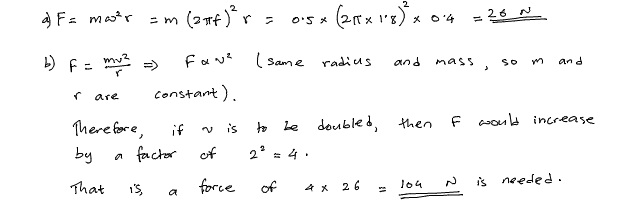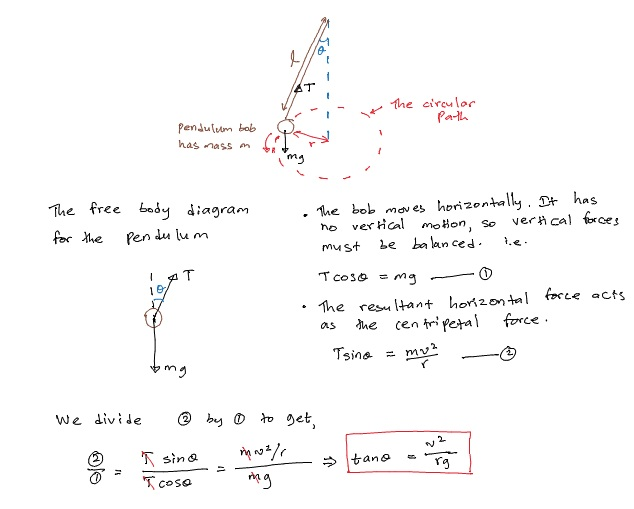# How to Calculate Centripetal Force

Before learning how to calculate centripetal force, let us see what is centripetal force and how it is derived. An object moving in a circular path is accelerating even if it is maintaining a constant speed. The acceleration experienced by such an object is called the centripetal acceleration, and it always points towards the centre of the circular path. According to Newton’s second law, there has to be a centripetal force pointing at the centre of the circular path, which is responsible for the circular motion. In this article, we look at several examples of how to calculate centripetal force.

## How to Find Centripetal Force

Deriving centripetal force is quite straightforward once you are familiar with the concepts of centripetal acceleration and Newton’s second law.

The centripetal acceleration on a body traveling at a constant speed$v$ in a circular path with a radius$r$ is given by$a=\frac{v^2}{r}$

If the angular speed of the body is$\omega$, then the centripetal acceleration could be written as$a={\omega^2}r$

Now, to go from centripetal force to centripetal acceleration, we simply make use of Newton’s second law of motion$F=ma$. Then, centripetal acceleration$F$ for a body having mass$m$ is,$F=\frac{mv^2}{r}$ and,$F=m{\omega^2}r$

## How to Calculate Centripetal Force

Example 1

A small ball of mass 0.5 kg is attached to a string and it is whirled at a constant speed in a horizontal circle, which has a radius of 0.4 m. The circular motion of the ball has a frequency of  1.8 Hz.

a) Find the centripetal force.

b) Calculate how much force would be needed to move the ball in the same circle, but with twice the speed.How to Calculate Centripetal Force – Example 1

## Examples of Centripetal Force

We will now look at several situations where the concepts we’ve learned about circular motion are applicable. The key to solving these types of problem is to identify the circular path and then find the resultant force pointing towards the centre of the circular path. This resultant force is the centripetal force.

### Circular Motion of a Conical Pendulum

Suppose a mass$m$ attached to the end of a string of length$l$ made to move in a horizontal circle with radius$r$, such that the string makes an angle$\theta$ to the vertical. The situation is illustrated below:How to Calculate Centripetal Force – Conical Pendulum

It is important to note here that the pendulum cannot be swung in a horizontal circle with the string parallel to the ground. Gravity is always pulling the pendulum down, so there must always be a vertical force to balance this out. The vertical force must come from the tension, which acts along the string. Therefore, in order for tension to be able to balance the downward pull of weight, the pendulum’s string must always be at an angle to the ground.

### Circular Motion and Banking

Banking occurs when, for example, a car is traveling on a tilted track in a circular path or when a pilot deliberately angles an aircraft to maintain a circular path. The free body diagram for both cases look similar, so I will use just one diagram to find the centripetal force in both cases. The only difference is that the force named$U$ for the car is the reaction force between the car’s tyres and the road surface, whereas for the aeroplane,$U$ is the “Lift” force from the wings. In both cases,$m$ refers to the mass of the car/aeroplane.How to Calculate Centripetal Force – Banking

Example 2

A car is travelling at 20 m s-1 in a banked section of a road. If the radius of the horizontal circular path is 200 m, calculate the banking angle necessary to keep the car moving at this speed, without any friction between the tyres and the road.

If there is friction, it would contribute to centripetal force and the vehicle would be able to move at a greater speed. However, we’re assuming friction is 0 here (imagine a very slippery road).